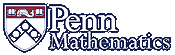Math 425: Partial Differential EquationsFaculty: Jerry L. Kazdan     Telephone: (215) 898-5109     email: kazdan AT math.upenn.edu     Office Hours: Wed. 10:30-11:30   (and also by appointment) in DRL 4E15 TA: Howard Levinson     Telephone: (412) 841-8097     email: levh AT sas.upenn.edu     Office Hours: ??? (and by appointment) in DRL 1N1

Partial Differential Equations, Spring 2015

Text: Walter A. Strauss, Partial Differential Equations: An Introduction, 2nd Edition, John Wiley (2007), ISBN-13: 9780470054567
As usual, since prices vary considerably, it is wise to search online for less expensive textbook sources.
Note that the first edition had many typos. For a list for both the first and second editions, see the author's web page
Content: The heart of this course is to achieve some real understanding of the wave, heat, and Laplace equations. The emphesis will be on mathematical and physical insight and ideas, not complicated formulas.
I taught this in Spring 2011. Although the course will be somewhat different, much of the material will be identical. You might find the homework, exams, and notes from that course useful: Math 425 Spring 2011

Some References: books, articles, web pages
Exams:

You may always use one 3"x5" card with handwritten notes on both sides

Notes:
LaTeX: If you will be writing many documents that contain equations, it is wise to learn (and use) LaTeX. It is available on Windows, Macs, and Linux -- and is free. See TeX Stuff. For some students, this might be the most useful item you learn in this course.
Some Classical PDEs
Striking a Match: Turbulence
Tacoma Narrows Bridge
Some notes (1965!) from a course like our Math 240 (there are typos.)
ODE's: Generalities on Linear ODE's   DeTurck Notes
DeTurck's Math 425 for 2010
DeTurck notes on first order PDE's
Derivation of the heat equation
PDE: Change Variables: print version   (display version)
Orthogonal Vectors and Fourier Series
Uniqueness for the initial value problem for the heat equation. The proof in Petrovsky assumes that the solution u(x,t) is bounded while the proof in John allows for the solution to grow at infinity as long as for any T there is a constant c so that |u(x,t)|< e(c|x|2) for all 0 ≤ t ≤ T. Note that if u(x,t) is allowed to grow too quickly at infinity, there are examples where uniqueness fails.
Standing Waves   Music
Gibbs Phenomenon
Lorentz Transformations
Sines and Cosines using ODE
Spherical Harmonics Spherical Harmonics (Wikipedia)
Spherical Harmonics: Strauss
Completeness of Eigenfunctions of the Laplacian. A more "geometric" version of the proof in Strauss, Sec 11.3.
Notes on Convolution
Hear the Shape of a Drum Google search on: Gordon "Shape of a drum"

JLK Australia 2008 Notes (for a slightly more advanced course)

Homework Assignments:

Old Exams: (you may always use one 3"x5" card with notes on both sides)
Spring 2011 Exam 1,     condensed,   (solutions).
Spring 2011 Exam 2,    condensed   (solutions)